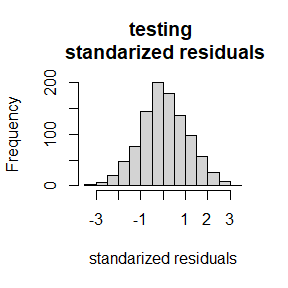# Getting started

## Overview

The goal of outerbase is to make the production of near-interpolators easy, stable, and scalable. It is based on a C++ backend and interfaced with R via {Rcpp}. Under the hood, it leverages unique, custom linear algebra using {RcppArmadillo} (Armadillo) and omp. The overall structure is designed to be interacted with in an object-oriented manner using Rcpp modules.

There are ways to interact with outerbase for those who are uncomfortable with (or just actively detest) object oriented programming.

library(outerbase)

Note that if you built this package from source, make sure you use a compiler that can process omp commands to access the entire speed benefits.

## Simple prediction

To understand how to get started with using the package, we predict using data from a function with an eight dimensional input commonly known as the Borehole function. We begin by generating 1000 points using a test function ?obtest_borehole8d built into the package.

sampsize = 400
d = 8
x = matrix(runif(sampsize*d),ncol=d) #uniform samples
y = obtest_borehole8d(x) + 0.5*rnorm(sampsize)

Our goal will be to design a predictor for y given x that is a near-interpolator.

The simplest way to interact with this package is using ?obfit (fitting outerbase). The function requires x, y and two other objects.

The value of numb is the number of basis functions you want to use. The choice of numb is still under research, but generally you want it to be large as tolerable. Play around!

The underlying concepts of this approach come from Gaussian processes. Thus the core building block of predictors will be covariances functions. The choice of the covariances needed for obfit is a list of strings corresponding to each column in x. Type listcov() to discover what covariance functions are are currently deployed.

listcov()
#>  "mat25pow" "mat25"    "mat25ang"

If you are curious about any of them, type, e.g. ?covf_mat25pow.

Note obfit has some checks in place to prevent serious damage. They are not foolproof.

obmodel = obfit(x, y, covnames=rep("elephant",8))
#> Error in .checkcov(covnames[k], x[, k]):
#>  covariances must be from listcov()
obmodel = obfit(x, y[1:200], covnames=rep("mat25pow",5))
#> Error in obfit(x, y[1:200], covnames = rep("mat25pow", 5)):
#>  x and y dims do not align
obmodel = obfit(x[1:2,], y[1:2], covnames=rep("mat25pow",8))
#> Error in obfit(x[1:2, ], y[1:2], covnames = rep("mat25pow", 8)):
#>  dimension larger than sample size has not been tested
obmodel = obfit(x, y, numb = 2, covnames=rep("mat25pow",8))
#> Error in obfit(x, y, numb = 2, covnames = rep("mat25pow", 8)):
#>  number of basis functions should be less than twice the dimension
obmodel = obfit(100*x, y, covnames=rep("mat25pow",8))
#> Error in .checkcov(covnames[k], x[, k]):
#>  x ranges exceed limits of covariance functions
#>  the limits are between 0 and 1
#>  try rescaling
obmodel = obfit(0.001*x, y, covnames=rep("mat25pow",8))
#> Error in .checkcov(covnames[k], x[, k]):
#>  x are too small for ranges
#>  the limits are between 0 and 1
#>  try rescaling

Below is one correct deployment, where mat25pow is used for all dimensions. This should take a bit to run, but it should be around a second on most modern computers.

ptm = proc.time()
obmodel = obfit(x, y, numb=300, covnames=rep("mat25pow",8),
verbose = 3)
#> doing partial optimization
#> max number of cg steps set to 100
#>
#> ########started BFGS#######
#>  iter.no obj.value wolfe.cond.1 wolfe.cond.2 learning.rate
#>        0   220.745           NA           NA           0.1
#>  iter.no obj.value wolfe.cond.1 wolfe.cond.2 learning.rate
#>        1    -296.9     -517.588     -133.116           0.4
#>  iter.no obj.value wolfe.cond.1 wolfe.cond.2 learning.rate
#>        2   -804.38      -507.41     -5558.19      0.109596
#>  iter.no obj.value wolfe.cond.1 wolfe.cond.2 learning.rate
#>        3   -1106.1     -301.688     -212.856      0.546858
#>  iter.no obj.value wolfe.cond.1 wolfe.cond.2 learning.rate
#>        4     -1177     -70.8663     -1377.63       0.29044
#>  iter.no obj.value wolfe.cond.1 wolfe.cond.2 learning.rate
#>        5  -1250.25      -73.245     -141.959      0.328663
#>  iter.no obj.value wolfe.cond.1 wolfe.cond.2 learning.rate
#>        6  -1297.93     -47.6694     -68.8574      0.367347
#>  iter.no obj.value wolfe.cond.1 wolfe.cond.2 learning.rate
#>        7  -1320.23     -22.3002     -24.9251       0.40604
#>  iter.no obj.value wolfe.cond.1 wolfe.cond.2 learning.rate
#>        8  -1336.55     -16.3209     -11.4459      0.444336
#>  iter.no obj.value wolfe.cond.1 wolfe.cond.2 learning.rate
#>        9  -1353.65     -17.0936      -12.776      0.481882
#>  iter.no obj.value wolfe.cond.1 wolfe.cond.2 learning.rate
#>       10  -1367.47     -13.8251     -14.6767      0.518378
#>  iter.no obj.value wolfe.cond.1 wolfe.cond.2 learning.rate
#>       11  -1374.04      -6.5679     -10.1321      0.553582
#>  iter.no obj.value wolfe.cond.1 wolfe.cond.2 learning.rate
#>       12  -1376.13     -2.08782      -13.956      0.587305
#>  iter.no obj.value wolfe.cond.1 wolfe.cond.2 learning.rate
#>       13  -1378.24     -2.10842      -3.1903      0.619409
#> num iter: 14  obj start: 220.7445  obj end: -1378.609
#> final learning rate: 0.6497998
#> approx lower bound (not achieved): -1379.679
#> #########finished BFGS########
#>
#> doing optimization  1
#> max number of cg steps set to 315
#>
#> ########started BFGS#######
#>  iter.no obj.value wolfe.cond.1 wolfe.cond.2 learning.rate
#>        0  -1379.31           NA           NA        0.3249
#>  iter.no obj.value wolfe.cond.1 wolfe.cond.2 learning.rate
#>        1  -1381.85     -2.53389     -1.74958        0.3249
#>  iter.no obj.value wolfe.cond.1 wolfe.cond.2 learning.rate
#>        2  -1384.84     -2.99056     -3.72161      0.363559
#>  iter.no obj.value wolfe.cond.1 wolfe.cond.2 learning.rate
#>        3  -1386.12     -1.28781     -2.39216       0.40227
#> num iter: 4  obj start: -1379.311  obj end: -1386.528
#> final learning rate: 0.4406213
#> approx lower bound (not achieved): -1387.874
#> #########finished BFGS########
#>
#> doing optimization  2
#> max number of cg steps set to 316
#>
#> ########started BFGS#######
#>  iter.no obj.value wolfe.cond.1 wolfe.cond.2 learning.rate
#>        0  -1386.34           NA           NA      0.220311
#>  iter.no obj.value wolfe.cond.1 wolfe.cond.2 learning.rate
#>        1  -1386.37   -0.0320092   -0.0255303      0.220311
#>  iter.no obj.value wolfe.cond.1 wolfe.cond.2 learning.rate
#>        2  -1386.39   -0.0267811   -0.0183269       0.25629
#>  iter.no obj.value wolfe.cond.1 wolfe.cond.2 learning.rate
#>        3  -1386.42   -0.0240469   -0.0264348      0.293669
#> num iter: 4  obj start: -1386.336  obj end: -1386.43
#> final learning rate: 0.3319501
#> approx lower bound (not achieved): -1386.475
#> #########finished BFGS########
print((proc.time() - ptm))
#> elapsed
#>    0.59

Note that the package is made using custom parallelization at the linear-algebra level. The package relies on omp for parallelization, so if the package was not compiled with that in place there will be no benefits. The default call of obfit grabs all available threads, which is ideal for desktops/laptops. It might be less ideal for large clusters where the CPU might be shared.

We can adjust the number of threads manually. Below we reduce ourselves to a single thread, which should slow things down.

ptm = proc.time()
obmodel = obfit(x, y, numb=300, covnames=rep("mat25pow",8),
print((proc.time() - ptm))
#> elapsed
#>    1.21

We can then predict using ?obpred. While it is not an exact interpolator, it is close.

predtr = obpred(obmodel, x)
rmsetr = sqrt(mean((y-predtr$mean)^2)) plot(predtr$mean, y,
main=paste("training \n RMSE = ", round(rmsetr,3)),
xlab="prediction", ylab = "actual")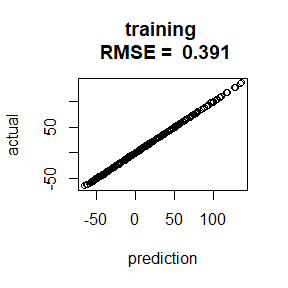Since we generated this data, we can show that outerbase can reasonably predict ground truth, meaning overfitting is not an issue.

ytrue = obtest_borehole8d(x)
rmsetr = sqrt(mean((ytrue-predtr$mean)^2)) plot(predtr$mean, ytrue,
main=paste("oracle \n RMSE = ", round(rmsetr,3)),
xlab="prediction", ylab="actual")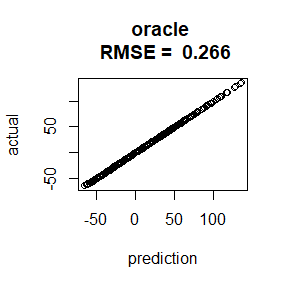1000 test points generated the same way as our original data can also serve as a verification process.

xtest = matrix(runif(1000*d),ncol=d) #prediction points
ytest = obtest_borehole8d(xtest) + 0.5*rnorm(1000)

The predictions at these new points are also quite good. Not quite as good as the residuals on the test set, but we are extrapolating here.

predtest = obpred(obmodel, xtest)

rmsetst = sqrt(mean((ytest-predtest$mean)^2)) plot(predtest$mean, ytest,
main=paste("testing \n RMSE = ", round(rmsetst,3)),
xlab="prediction", ylab="actual")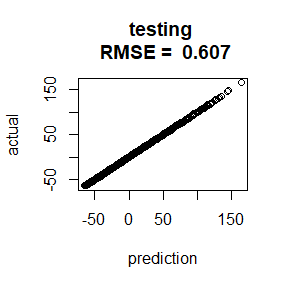This package also produces variances on the predictions which we can use to test reasonableness. The fact that the second histogram looks like a standard Normal is promising that the predictions are reasonable.

hist((ytest-predtest$mean), main="testing \n residuals", xlab="residuals")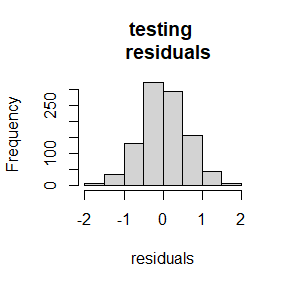hist((ytest-predtest$mean)/sqrt(predtest\$var),
main="testing \n standarized residuals",
xlab="standarized residuals")